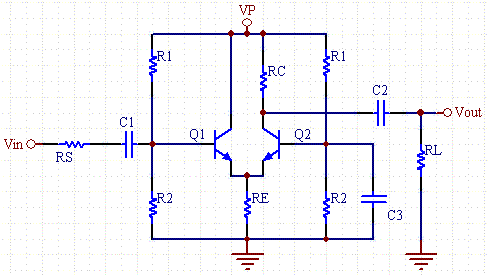# Wide-band Differential Amplifier Calculator

The wide-band differential amplifier has high gain and high band width. It overcomes the Miller capacitance limitations of the common emitter amplifier by tying the collector of Q1, and the base of Q2 to signal ground. As a result it can achieve gain bandwidths orders of magnitude larger than the common emitter amplifiers. The only cost is that a second transistor is needed.This calculator computes bias voltage and current levels, as well as gain and frequency response.

 R1 (K ohms) R2 (K ohms) RC (Collector resistor) (K ohms) RE (Emitter resistor) (K ohms) VP (Supply Voltage) (V) Beta (DC Current Gain) VBE (Base to emitter drop) Rs (Source Resistance): (ohms) RL (Load resistor) (ohms) fT (Current Gain BW Product): (MHz) CCB (Cu Collector-Base Cap.): (pF) CBE (Cπ, Base-Emitter Cap.): Optional (pF) VC (Collector Voltage) (V) VE(Emitter Voltage) (V) VB (Base Voltage) (V) IE (Emitter Voltage) (mA) IC (Collector Voltage) (mA) IB (Base Voltage) (mA) gm (Transconductance) rπ (Input Resistance of BJT at low freq) (ohms) re (ohms) RIN(Input Resistance of Amp) (K ohms) A (Amplifier Voltage Gain) fP1 (MHz) fP2 (MHz)

### Equations

VB= VP*R2/(R1+R2)

VE= VB-VBE

IE=VE/RE

Alpha= Beta/(Beta+1);

IC= Alpha*IE

VC= VP - IC*RC/2

IB=IC/Beta

gm = Ic/25mA

re= Alpha/gm

rπ=Beta/gm

A=Alpha* rπ*RC/(Rs+2*rπ)/re

fP1=1/(2*π*(Rs||2*rπ)*(CBE/2+CBC))

fP2=1/(2*π*RC*CBC)

fT=gm/(2π*(CBE+CBC))

The lower pole is dominate.